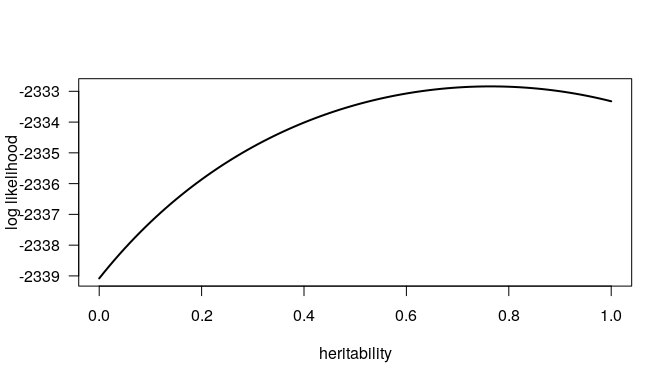R/lmmlite user guide

R/lmmlite is an R package for fitting linear mixed models in the context of genome-wide association studies (GWAS) and quantitative trait locus (QTL) mapping. At present, it is not intended for “production” use. I wrote this to try to get a better understanding of how to fit these models, and I’ve not yet put any effort into checking arguments and omitting cases with missing values.

The code closely follows Nick Furlotte’s pylmm.

The sort of model I want to fit is $$y = X\beta + \epsilon$$ where $$\epsilon$$ is multivariate normal with mean 0 and variance $$\sigma^2_g K + \sigma^2_e I$$, where $$K$$ is a known kinship matrix and $$I$$ is the identity. In pylmm, the focus is on $$\sigma^2 = \sigma^2_g + \sigma^2_e$$ and the heritability $$h^2 = \sigma^2_g / \sigma^2$$.

We’ll focus here on the recla dataset that I include with the package. This is data on a set of diversity outcross mice, from Recla et al. (2014) and Logan et al. (2013). The data are originally from the QTL Archive/Mouse Phenotype Database and I’ve also placed them online in the format used by R/qtl2. I used qtl2geno to calculate genotype probabilities from the MUGA SNP array data. The data include 261 mice, and form a list with three components: the estimated kinship matrix, a matrix with 26 phenotypes, and a covariate matrix that contains an intercept (all 1’s) and sex (0 for female and 1 for male).

library(lmmlite)
data(recla)

The package includes two implementations of the fit of linear mixed models (LMMs): plain R, and C++ (using the Rcpp and RcppEigen packages, the latter being an interface to the Eigen C++ linear algebra library).

R/lmmlite includes just four functions: eigen_rotation(), getMLsoln(), calcLL(), and fitLMM(). In each case, use use_cpp=FALSE to use the plain R implementation and use_cpp=TRUE (the default) to use the C++ implementation.

The first function, eigen_rotation(), calculates the eigen decomposition of the kinship matrix and “rotates” the phenotypes and covariates by pre-multiplying them with the tranpose of the matrix of eigenvectors. This rotation greatly simplifies later calculations by turning a general least squares problem into a weighted least squares problem.

Care must be taken with missing values in the phenotypes and covariates. In practice, one must work with batches of phenotypes that have a common missing data pattern, and first remove individuals with missing values. Here, we’ll focus on the first phenotype.

e <- eigen_rotation(recla$kinship, recla$pheno[,1], recla$covar) The output is a list containing four components: Kva is the vector of eigenvalues of the kinship matrix, Kva_t is the transposed matrix of eigenvectors, y is the rotated phenotype matrix, and X is the rotated covariate matrix. The next function getMLsoln() will estimates the coefficients ($$\beta$$) and residual variance ($$\sigma^2 = \sigma^2_g + \sigma^2_e$$) for a given value of the heritability ($$h^2 = \sigma^2_g / \sigma^2$$). It takes, as input, a single value for the heritability, and then Kva, y, and X, as returned from eigen_rotation(). A further argument reml, indicates whether REML will later be used, in which case the log determinant of the matrix $$X' W X$$, where $$W$$ is a diagonal matrix of weights, is calculated. ml_soln <- getMLsoln(0.5, e$Kva, e$y, e$X)

The result is a list containing beta and sigmasq, with the residual sum of squares (RSS) and log det($$X' W X$$) included as attributes.

The function calcLL() calculates the log likelihood for a given value of the heritability. (It can also take a vector of heritabilities.) It takes the same arguments as getMLsoln(), and in practice one would probably not call getMLsoln() directly.

hsq <- seq(0, 1, by=0.01)
ll <- calcLL(hsq, e$Kva, e$y, e$X) We can plot the results as follows. plot(hsq, ll, type="l", lwd=2, las=1, xlab="heritability", ylab="log likelihood")If you call calcLL() with a single heritability value, the results include the estimates of beta and sigmasq as attributes. one_ll <- calcLL(0.5, e$Kva, e$y, e$X)
attr(one_ll, "beta")
##  1413.98691  -28.17931
attr(one_ll, "sigmasq")
##  276158.5

The final function, fitLMM(), optimizes the log likelihood to estimate the heritability. The result is a list containing beta, sigmasq, hsq, sigmasq_g, sigmasq_e, and loglik.

(out <- fitLMM(e$Kva, e$y, e$X)) ##$beta
##  1417.98330  -23.24218
##
## $sigmasq ##  295340.5 ## ##$hsq
##  0.7642841
##
## $sigmasq_g ##  225724.1 ## ##$sigmasq_e
##  69616.46
##
## \$loglik
##  -2332.84

The fitLMM() function includes an argument check_boundary; if TRUE (the default), the 0 and 1 boundaries are checked explicitly, in seeking to estimate the heritability.